Courses

# Electrochemistry MCQ - 2 (Advanced)

## 15 Questions MCQ Test Chemistry for JEE Advanced | Electrochemistry MCQ - 2 (Advanced)

Description
This mock test of Electrochemistry MCQ - 2 (Advanced) for JEE helps you for every JEE entrance exam. This contains 15 Multiple Choice Questions for JEE Electrochemistry MCQ - 2 (Advanced) (mcq) to study with solutions a complete question bank. The solved questions answers in this Electrochemistry MCQ - 2 (Advanced) quiz give you a good mix of easy questions and tough questions. JEE students definitely take this Electrochemistry MCQ - 2 (Advanced) exercise for a better result in the exam. You can find other Electrochemistry MCQ - 2 (Advanced) extra questions, long questions & short questions for JEE on EduRev as well by searching above.
*Multiple options can be correct
QUESTION: 1

### In which of the following cell (s) : E cell = Ecell° ?

Solution:
*Multiple options can be correct
QUESTION: 2

### Which one is/are correct among the following?Given, the half cell emf’s E°Cu+2|Cu = 0.337, E°Cu+1|Cu = 0.521

Solution: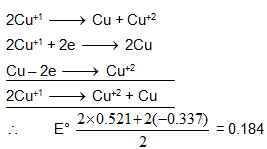*Multiple options can be correct
QUESTION: 3

### Indicate the correct statements:

Solution:
*Multiple options can be correct
QUESTION: 4

The standard redox potential E° of the following systems are SystemE° (volts)

(i) MnO4- + 8H+ + 5e- → Mn2+ + 4H2O 1.51

(ii) Sn2+→ Sn4+ + 2e- - 0.15

(iii) Cr2O72- + 14H+ + 6e- → 2Cr3+ + 7H2O 1.33

(iv) Ce3+ → Ce4+ + e- - 1.61 The oxidizing power of the various species are related as

Solution:

the SRP should be high for a species to be a good oxidizing agent.

QUESTION: 5

A fuel cell is a cell that is continuously supplied with an oxidant and a reductant so that it can deliver a current indefinitely.
Fuel cells offer the possibility of achieving high thermodynamic efficiency in the conversion of Gibbs energy into mechanical work. Internal combustion engines at best convert only the fraction (T2 – T1)/T2 of the heat of combustion into mechanical work.

While the thermodynamic efficiency of the fuel cell is given by,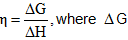is the
Gibbs energy change for the cell reaction and Δ H is the enthalpy change of the cell reaction.
A hydrogen-oxygen fuel cell may have an acidic or alkaline electrolyte.

Pt|H2(g)|H+(aq.)||H2O( l )|O2(g)|Pt;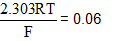The above fuel cell is used to produce constant current supply under constant temperature & 30 atm constant total pressure condition in a cylinder. If 10 moles H2 and 5 moles of O2 were taken initially. Rate of combustion of O2 is 10 milli moles per minute. The half cell reactions are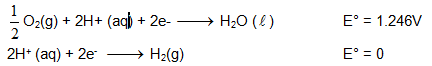To maximize the power per unit mass of an electrochemical cell, the electronic and electrolytic resistances of the cell must be minimized. Since fused salts have lower electrolytic resistances than aqueous solutions, high-temperature electrochemical cells are of special interest for practical applications.

Calculate e.m.f. of the given cell at t = 0. (log 2 = 0.3).

Solution: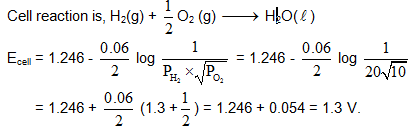QUESTION: 6

A fuel cell is a cell that is continuously supplied with an oxidant and a reductant so that it can deliver a current indefinitely.
Fuel cells offer the possibility of achieving high thermodynamic efficiency in the conversion of Gibbs energy into mechanical work. Internal combustion engines at best convert only the fraction (T2 – T1)/T2 of the heat of combustion into mechanical work.

While the thermodynamic efficiency of the fuel cell is given by,is the
Gibbs energy change for the cell reaction and Δ H is the enthalpy change of the cell reaction.
A hydrogen-oxygen fuel cell may have an acidic or alkaline electrolyte.

Pt|H2(g)|H+(aq.)||H2O( l )|O2(g)|Pt;The above fuel cell is used to produce constant current supply under constant temperature & 30 atm constant total pressure condition in a cylinder. If 10 moles H2 and 5 moles of O2 were taken initially. Rate of combustion of O2 is 10 milli moles per minute. The half cell reactions areTo maximize the power per unit mass of an electrochemical cell, the electronic and electrolytic resistances of the cell must be minimized. Since fused salts have lower electrolytic resistances than aqueous solutions, high-temperature electrochemical cells are of special interest for practical applications.

Q.

The above fuel cell is used completely as an electrolytic cell with Cu voltameter of resistance 26.94 W using Pt electrodes. Initially Cu voltameter contains 1 litre solution of 0.05M CuSO4.[H+] in solution after electrolysis (Assuming no change on volume of solution).

Solution: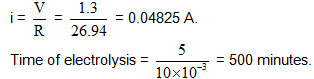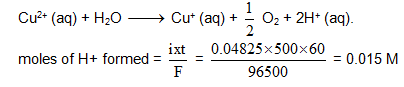QUESTION: 7

A fuel cell is a cell that is continuously supplied with an oxidant and a reductant so that it can deliver a current indefinitely.
Fuel cells offer the possibility of achieving high thermodynamic efficiency in the conversion of Gibbs energy into mechanical work. Internal combustion engines at best convert only the fraction (T2 – T1)/T2 of the heat of combustion into mechanical work.

While the thermodynamic efficiency of the fuel cell is given by,is the
Gibbs energy change for the cell reaction and Δ H is the enthalpy change of the cell reaction.
A hydrogen-oxygen fuel cell may have an acidic or alkaline electrolyte.

Pt|H2(g)|H+(aq.)||H2O( l )|O2(g)|Pt;The above fuel cell is used to produce constant current supply under constant temperature & 30 atm constant total pressure condition in a cylinder. If 10 moles H2 and 5 moles of O2 were taken initially. Rate of combustion of O2 is 10 milli moles per minute. The half cell reactions areTo maximize the power per unit mass of an electrochemical cell, the electronic and electrolytic resistances of the cell must be minimized. Since fused salts have lower electrolytic resistances than aqueous solutions, high-temperature electrochemical cells are of special interest for practical applications.

Q.

If λm (Cu2+) = 0.01S m2 mole -1, λm (H+) = 0.035 S m2 mole-1 and λm (SO42-) = 0.016 S m2 mole-1, specific conductivity of resulting solution left in sopper voltameter after above electrolysis is

Solution:

In resulting solution, [Cu2+] = 0.0075 = 0.0425 M, [H+] = 0.015 M and [SO42- = 0.05 M.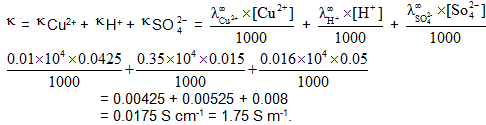QUESTION: 8

Titrations are one of the methods we can use to discover the precise concentrations of solution. A typical titration involves adding a solution from a burette to another solution in a flask. The endpoint of the titration is found by watching a colour change taking place. However, a problem arises when a suitable indicator cannot be found, or when the colour changes involved are unclear. In cases redox potential may sometimes come to the rescue.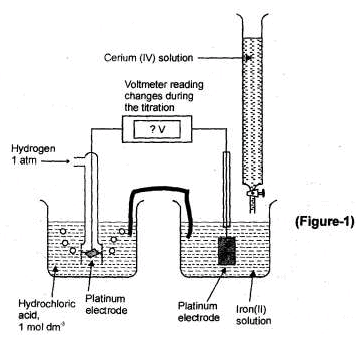A particularly well known example (Fig.1) is a method of discovering the concentration of iron in a solution by titrating them with a solution of cerium (IV). The redox potential that are

of interest here are E°Fe3+/Fe2+ = + 0.77 V and E°Ce4+/Ce3+ = + 1.61 V. These tell us that cercium (IV) ions are the oxidizing agents, and iron (II) ions are the reducing agent. They should react according to the equation

Fe2+ (aq)  + Ce4+ (aq) → Fe3+ (aq) + Ce3+ (aq)

Now imagine that we know the concentration of the cerium (IV) ions solution in the burette. We want to measure the concentration of the iron (II) solution. If we add just one drop of the cerium (IV) solution from the bruette, some of the iron (II) ions will be oxidised. As a consequence the beaker would now contain a large number of unreacted ions, but also some iron (III) ions as well. All of the cerium (III). The solution in the beaker now represents an iron(III)/iron(II) half cell, although not at standard conditions. Thus the e.m.f. of the cell will be near, but not equal, to E°Fe3+/Fe2+.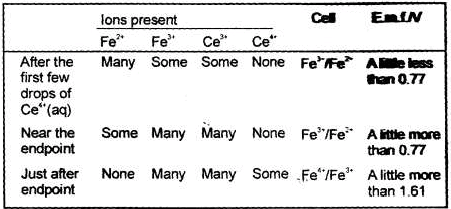to ad cerium (IV) solution, the number of iron (II) ions is gradually reduced and eventually only a very few are left (Table).At this stage the next few drops of cerium (IV) solution convert all the remaining iron (II) ions into iron (III), and some of the cerium (IV) ions are left unreacted. Once this happens we no longer ions and a smaller number of cerium (IV) ions. The solution in the beaker now behaves as a cerium (IV)/cerium (III) half-cell (although not a standard one).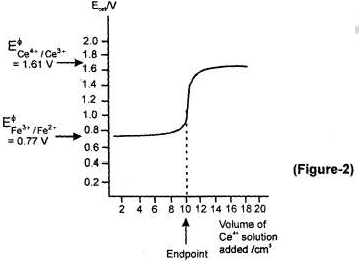Just before all the iron (II) ions are converted into iron (III) we have a cell with an e.m.f.of around + 0.77 V. After all the iron (II) ions are oxidised, we have a cell with an e.m.f. of about + 1.61 V. This rapid rise in e.m.f. occurs with the addition of hust one drop of cerium (IV) solution. You should be able to understand why a graph of cell e.m.f. against volume of

cerium (IV) solution added looks like that of Fig. 2. The end point of the titration can be read from the graph and the concentration of the iron (II) solution calculated in the usual way

Q.

When an ion is converted into a complex ion, the redox potential changes. You can see this in the case of the e.m.f. of the iron (III)/ iron (II) system (+ 0.77 V) and the hexacyanoferrate (III)/ hexacyanoferrate (II) system (+0.36 V). The cyanide ion is said to stabilize the oxidation state of the iorn. If you were to make up a cell What would be the e.m.f. and what would be the cell reaction?

Solution: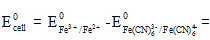+ 0.77 V – 0.36 V = + 41 V

The reaction is: Fe (CN)6 4- (aq) + Fe3+ (aq) → Fe(CN)6 3- (aq) + Fe2+ (aq)

QUESTION: 9

Titrations are one of the methods we can use to discover the precise concentrations of solution. A typical titration involves adding a solution from a burette to another solution in a flask. The endpoint of the titration is found by watching a colour change taking place. However, a problem arises when a suitable indicator cannot be found, or when the colour changes involved are unclear. In cases redox potential may sometimes come to the rescue.A particularly well known example (Fig.1) is a method of discovering the concentration of iron in a solution by titrating them with a solution of cerium (IV). The redox potential that are

of interest here are E°Fe3+/Fe2+ = + 0.77 V and E°Ce4+/Ce3+ = + 1.61 V. These tell us that cercium (IV) ions are the oxidizing agents, and iron (II) ions are the reducing agent. They should react according to the equation

Fe2+ (aq)  + Ce4+ (aq) → Fe3+ (aq) + Ce3+ (aq)

Now imagine that we know the concentration of the cerium (IV) ions solution in the burette. We want to measure the concentration of the iron (II) solution. If we add just one drop of the cerium (IV) solution from the bruette, some of the iron (II) ions will be oxidised. As a consequence the beaker would now contain a large number of unreacted ions, but also some iron (III) ions as well. All of the cerium (III). The solution in the beaker now represents an iron(III)/iron(II) half cell, although not at standard conditions. Thus the e.m.f. of the cell will be near, but not equal, to E°Fe3+/Fe2+.to ad cerium (IV) solution, the number of iron (II) ions is gradually reduced and eventually only a very few are left (Table).At this stage the next few drops of cerium (IV) solution convert all the remaining iron (II) ions into iron (III), and some of the cerium (IV) ions are left unreacted. Once this happens we no longer ions and a smaller number of cerium (IV) ions. The solution in the beaker now behaves as a cerium (IV)/cerium (III) half-cell (although not a standard one).Just before all the iron (II) ions are converted into iron (III) we have a cell with an e.m.f.of around + 0.77 V. After all the iron (II) ions are oxidised, we have a cell with an e.m.f. of about + 1.61 V. This rapid rise in e.m.f. occurs with the addition of hust one drop of cerium (IV) solution. You should be able to understand why a graph of cell e.m.f. against volume of

cerium (IV) solution added looks like that of Fig. 2. The end point of the titration can be read from the graph and the concentration of the iron (II) solution calculated in the usual way

Q.

Imagine you were given a solution of potassium dichromate (VI) in a beaker, and a solution of iron (II) sulphate in a burette. You do not know the concentration of dichromate (VI) ions, but the concentration of the iron (II) solution is known. Your task is to carry out a redox titration using the two solutions in order to determine the concentration of dichromate(VI) ions. Sketch a graph how the e.m.f. changes in the course of above titration. E° Cr2O2-7/Cr3+ = 1.33 V, E°Fe3+/Fe2+ = 0.77 V.

Solution:

After one drop iron (II) solution is added the beaker will contain a mixture of Cr2O72-, Cr3+ and Fe3+ ions. The e.m.f. will be near to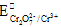= + 0.77. The reaction is:

6Fe2+ (aq) + Cr2O72- (aq) + 14H+ (aq) → 6Fe3+ (aq) + 2Cr3+ (aq) + 7H2O(I)

The apparatus would be like that in figure. The graph is shown is figure.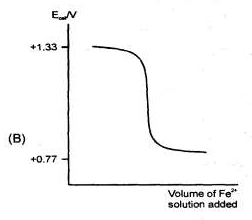QUESTION: 10

Titrations are one of the methods we can use to discover the precise concentrations of solution. A typical titration involves adding a solution from a burette to another solution in a flask. The endpoint of the titration is found by watching a colour change taking place. However, a problem arises when a suitable indicator cannot be found, or when the colour changes involved are unclear. In cases redox potential may sometimes come to the rescue.A particularly well known example (Fig.1) is a method of discovering the concentration of iron in a solution by titrating them with a solution of cerium (IV). The redox potential that are

of interest here are E°Fe3+/Fe2+ = + 0.77 V and E°Ce4+/Ce3+ = + 1.61 V. These tell us that cercium (IV) ions are the oxidizing agents, and iron (II) ions are the reducing agent. They should react according to the equation

Fe2+ (aq)  + Ce4+ (aq) → Fe3+ (aq) + Ce3+ (aq)

Now imagine that we know the concentration of the cerium (IV) ions solution in the burette. We want to measure the concentration of the iron (II) solution. If we add just one drop of the cerium (IV) solution from the bruette, some of the iron (II) ions will be oxidised. As a consequence the beaker would now contain a large number of unreacted ions, but also some iron (III) ions as well. All of the cerium (III). The solution in the beaker now represents an iron(III)/iron(II) half cell, although not at standard conditions. Thus the e.m.f. of the cell will be near, but not equal, to E°Fe3+/Fe2+.to ad cerium (IV) solution, the number of iron (II) ions is gradually reduced and eventually only a very few are left (Table).At this stage the next few drops of cerium (IV) solution convert all the remaining iron (II) ions into iron (III), and some of the cerium (IV) ions are left unreacted. Once this happens we no longer ions and a smaller number of cerium (IV) ions. The solution in the beaker now behaves as a cerium (IV)/cerium (III) half-cell (although not a standard one).Just before all the iron (II) ions are converted into iron (III) we have a cell with an e.m.f.of around + 0.77 V. After all the iron (II) ions are oxidised, we have a cell with an e.m.f. of about + 1.61 V. This rapid rise in e.m.f. occurs with the addition of hust one drop of cerium (IV) solution. You should be able to understand why a graph of cell e.m.f. against volume of

cerium (IV) solution added looks like that of Fig. 2. The end point of the titration can be read from the graph and the concentration of the iron (II) solution calculated in the usual way

Q.

The cell shown below was set up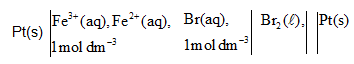What would be the cell e.m.f.? If potassium cyanide solution were added to the left hand half cell (with due care!), what would you expect to happen to the e.m.f. of the cell? E° Br2/Br- = 1.07V and use data of previousquestion, if required.

Solution: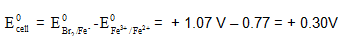If cyanide ions are added, the left hand half cell would change its e.m.f. to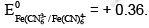Therefore the e.m.f. would change to + 0.71V.

QUESTION: 11

The molar conductance of NaCl varies with the concentration as shown in the following table.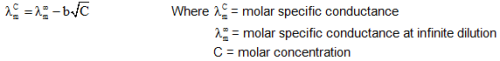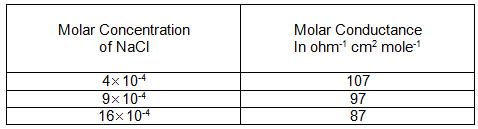When a certain conductivity cell (C) was filled with 25 ´ 10-4(M) NaCl solution. The resistance of the cell was found to be 1000 ohm. At infinite dilution, conductance of Cl - and SO4–2 are 80 ohm-1cm2mole-1 and 160 ohm-1cm2mole-1 respectively.

Q.

What is the molar conductance of NaCl at infinite dilution?

Solution: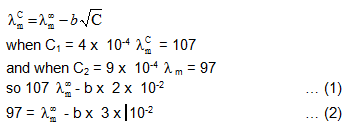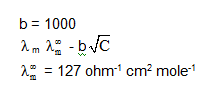QUESTION: 12

The molar conductance of NaCl varies with the concentration as shown in the following table.When a certain conductivity cell (C) was filled with 25 ´ 10-4(M) NaCl solution. The resistance of the cell was found to be 1000 ohm. At infinite dilution, conductance of Cl - and SO4–2 are 80 ohm-1cm2mole-1 and 160 ohm-1cm2mole-1 respectively.

Q.

What is the cell constant of the conductivity cell (C)

Solution: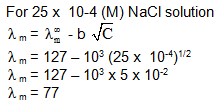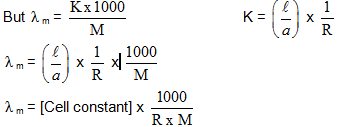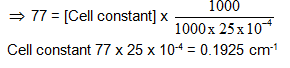QUESTION: 13

The molar conductance of NaCl varies with the concentration as shown in the following table.When a certain conductivity cell (C) was filled with 25 ´ 10-4(M) NaCl solution. The resistance of the cell was found to be 1000 ohm. At infinite dilution, conductance of Cl - and SO4–2 are 80 ohm-1cm2mole-1 and 160 ohm-1cm2mole-1 respectively.

Q.

If the cell (C) is filled with 5 ´ 10-3 (N) Na2SO4 the  observed resistance was 400 ohm. What is the molar conductance of Na2SO4.

Solution:

For Na2SO4 solution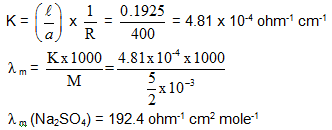QUESTION: 14

Match Matrix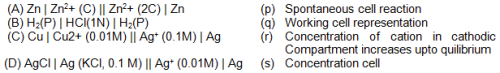Solution:

Based on facts.

QUESTION: 15

Match the following: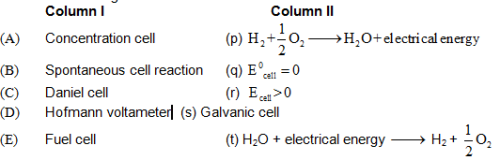Solution:

Factual question